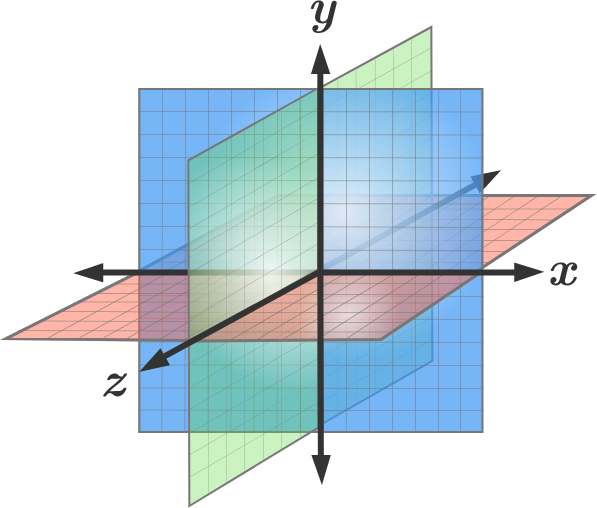Geometry

# 3D Coordinate Geometry: Level 3 Challenges

What is the shortest distance of the plane $4x - 3y + 12 z= 78$ from the origin in $\mathbb{R}^{3}$?In the $xyz$ coordinates, the centroids of the 3 faces of a cuboid are located at points $(2 , 2 , 2), (7 , 5 , 2)$, and $(7 , 2 , 4)$, where each side is parallel to one of the axes.

What is the volume of this cuboid?

Let $T$ be the triangle with vertices $(14, 0, 0)$, $(0, 21, 0)$, and $(0, 0, 42)$.

Line $\ell$ goes through the origin $O$ and intersects $T$ perpendicularly in point $P$.

Determine the distance $OP$.The graph $x^2 + y^2 = \dfrac{z^2}{3}$ intersects with the graph $z = ky + c$, where $k$ and $c$ are real numbers, such that the intersected points form a parabola with the point $\left (0 , \dfrac{-1}{2}, \dfrac{\sqrt{3}}{2}\right)$ as its vertex.

What is the value of $k^2$?

Find the coordinates of the point where the line through $(5, 1, 6)$ and $(3, 4, 1)$ crosses the $YZ$-plane

Submit answer as the sum of the coordinates.

×